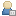Show Posts

This section allows you to view all posts made by this member. Note that you can only see posts made in areas you currently have access to.

Topics - SERGIO_ManOwaR_

Pages: 
1
Purebasic / [GAME] PureBasic SOKOBAN
« on: March 23, 2019 »
Hello! I want to share this sokoban i've coded for purebasic. Yep, it's just another sokoban, nothing special.

https://mega.nz/#!QKAFzYTA!avOYfPsetUDxmBJ2CdA7TFS9WgJUAZpLA80Ma86OnYs

INCLUDES:
* Over 2 thousand levels in 12 collections.
* Classic controls UNDO, QUICK SAVE AND QUICK LOAD.
* Nice engine with zooming to fit the screen and scrolling if the level is too large.
* Several graphic skins with static or animated backgrounds.
* Records auto-saved to file.

-I haven't created the levels nor skins. Info about levels and credits are included.
-Game music and SFX is OFF. Use [M] and [X] to turn ON

And not much... the game is no more under development because i've lost the main project source. I still have the "include file" sources if any wants to chek i'll share but any changes on the sources has no effect since game it's already compiled.

Hope it works and have fun! Sokoban is a very addictive game and some levels are great, the microban collections by David W. Skinner are superfun and many others.

2
Purebasic / PLATOO! A tiro skeet game (Demo)
« on: September 07, 2017 »
Hello! This is my presentation to purebasic forum.
I've been coding for yabasic so many years and recently discovered purebasic and give it a try... and soon started to code demos and stuff... it's a good step from yabasic.
I will like to share this little demo of a game started to develop at the same time learning languaje so it's simple but works ok. It's called PLATOO! and it's about shooting skeet.

Unfortunately i've lost latest versions and source code so i decided to share the latest .exe i have from the game, before to start building levels and objectives and even before i got a clear idea of what to do with this game.
It basically consist in the main loop where you can shoot skeets until out of ammo in a few random escenaries.
No objetives nor game progression sadly, but you can beat the hi score for each escenary and records will be saved to file.

Controls are mouse/keyboard or gamepad. If default gamepad controls don't satisfy you can edit the joystick pressing [J] in game. (Changes will be saved to file and loaded automatically next time if gamepad plugged)

Let's go for the important INFO:

OPTIONS:
* Languajes: You can choose SPANISH/ENGLISH at the very first screen of the game. Result will be saved to file.
* Controls: You can use mouse/keyboard or a gamepad. If you want to use gamepad plugg it before game or
change escenary to autodetect. Move analog sticks to all directions before play to detect the sticks's limits.
Personalice joystick pressing [J]. Changes will be saved to file.

CONTROLS:
* IN GAME:
-MOUSE LEFT=SHOT
-[INTRO]=PAUSE
-[ESC]=EXIT GAME/REPLAY
-[C]=Continue (to next random escenary)
-[W]=Watch instant replay (after play)
-[R]=Repeat current escenary
-,=Select spanish/english in real time
You can delete a record after beated it [H], also you can delete the records.dat file and all records will be
cleared

OS:
* Windows 32 bit (it may work on 64 bit or maybe not i dunno)

PERFORMANCE:
* Game intended to run at 60 FPS. You can chek FPS by pressing [RIGHT CAPS]+[F]
* Resolution: It works in 600*400. If your screen doesn't support will auto switch to standard 640*480

FEATURES:
* Give it a try!! I think it's nice game, simple and effective.
* Included some awesome .wav tunes from movies and shows probably well knowed.
* Any changes on config, controls or records will be saved to same folder attached to .exe so i suggest to keep
PLATOO.exe in a folder.

PROBLEMS RESOLUTION:
* WARNING! Suspend pc or exit fullscreen mode may cause game crash or stucked. If you can't return to
fullscreen mode you probably need to terminate .exe [CTRL]+[CAPS]+[ESC] I've tryed to follow instructions of

THAT'S ALL FOLKS! Hope enjoy this little game... and comments are welcome!

https://mega.nz/#F!YLYGyToT!g9MSL-t6-w0uwXZjrB05WA

3
Yabasic / GAME: FLAPPY YAB-REX
« on: November 23, 2015 »
Hi everybody, YABASIC lovers!
I've been missing out of forum so long, but just read something about a ps2 yabasic implementation for javascript... that sounds like great news and i'll keep an eye on it.
Meanwhile, i would like to share a game wich supposed to be a clone of google chrome's easter egg dino game. (https://www.youtube.com/watch?v=gymfJGSBErE)
It's a very simple game and i thought can be a good game to practice some coding...
After some days.. started to add some more stuff... new obstacles... new movements... and last added a nice zooming graphic engine... so, the result is a very nice game easy to play.
Gameplay is quite boring after a while, since there is no levels;just coin-op arcade style, more and more random obstacles until you crash...
STILL CODING FOR YABASIC IN 2015! Is there anybody more?

Note:Game is designed to run at 60FPS. Since emulator's default config is 50FPS you must
configure emu for smooth  graphics. (Details in source code)

HOPE ENJOY!

4
Yabasic / YA-GOLF MINI Super challengue
« on: July 10, 2012 »
Hi everybody!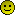After so many hours of working, I've decided to share this game with all.
I't a mini golf game with aerial view.

You can download or just select and copy the source code to the clipboard and paste it directly in the emulator or in a text file (or create your .P2Y extension file)
FILE SIZE IS 591 KB So it may take a few seconds to copy.

Game looks great in the emulator and finally could implement some bitmaps and simple but nice graphics, and my particullary tribute to a dear friend in a intro screen.

---------------------------------
GAME SPECS

Game Versión is 0.5.1 (This version has a bug in motion replay, plese download another one)

Yet some bugs and much work to do but most of the problems were fixed and game runs smooth in most Pcs with reasonable perfomance.

8 Players can be selected for each game, and controls can be settled HUMAN OR CPU

There are 2 FIELDS AVALIABLE. (1 working progress)

Game includes some routines to control flush rate acording your pc's performance. (Hope it works for everybody)

Controls info and some help included in-game, especially how to configure the
ps2 emulator to run it.

Some debug options can be settled in the source code; disable intro screen and other screens and some more configurable options (remarks are in spanish, ask if you want)
---------------------------------

---------------------------------
EMULATOR.

You need the win32 ps2 yabasic emulator to run it. (avaliable in this link)
http://members.iinet.net.au/~jimshaw/Yabasic/ps2yabasic1.6b3.zip

¡¡¡¡¡¡¡¡¡¡¡¡¡¡¡¡¡¡¡¡¡¡¡¡¡¡¡¡¡¡¡¡¡¡¡¡¡¡¡¡¡¡¡¡¡¡¡¡¡¡¡¡¡¡¡¡¡¡¡¡¡¡¡¡¡¡¡¡¡¡¡¡¡¡¡¡¡¡¡¡¡¡¡¡¡¡¡
WARNING! This game needs a especific configuration of the emulator
to work properly!

To set the right configuration config the emulator this way:
Win32 yabasic emulator>EDIT>OPTIONS>

SOURCE COLORING: OFF
NO SINC: ON
FORCE FLUSH RATE: OFF
¡¡¡¡¡¡¡¡¡¡¡¡¡¡¡¡¡¡¡¡¡¡¡¡¡¡¡¡¡¡¡¡¡¡¡¡¡¡¡¡¡¡¡¡¡¡¡¡¡¡¡¡¡¡¡¡¡¡¡¡¡¡¡¡¡¡¡¡¡¡¡¡¡¡¡¡¡¡¡¡¡¡¡¡¡¡¡

Future versions will be posted.. still so many work to do!!! (n' lots of bugs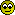)

Hope you like it!!! Yabasic is not dead and I would like to read some feedbacks.

With my greetings to all yabasic lovers and specially for Jimshaw for the great emulator.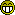-------------------------
VERSIONS HISTORY:
-------------------------

Vr 0.5.1:
First released version. Due to last hour changes it has some critical bugs.
The motion replay option dosn't work right and some others.

Vr 0.5.2: Quiqk fix for those bugs.

-----------------------------
-----------------------------

I Have modified the original post. My first will was to share the source code in this page to be copy/pasted for anybody even non-registered users, but source code is too long for the page and was cutted, so i've removed it.

5
Yabasic / 2D SCROLL DEMO
« on: July 10, 2012 »
Hi everybody!I'm  sharing this old ps2 demo  just to learn how to post at DBF forums where this is my first topic, so I hope everything goes right.

If there are still any people interested in YABASIC i would be pleased to continue sharing more code.
Workin' on some things which really looks great in the emu...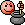This one was writed for the ps2 and I haven't adapted for the emulator; it is the original code
so it's not 100% compatible with the emu but it works.

Sorry for the crappy code! haha... by looking it now i realise its a completelly mess... from
the beginning to the end, no way to understand a word... it is the work of a  noob and
i'm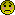to see it, but anyway, sharing cos' I really want to start sharing something.

------------------------------
CONTROLS:

Arrow keys: MOVEMENT. Keep presed on a block to push it (If allowed).
[Supr]: STOP MOVEMENT. Keep pressed to look forward.
[Ins]: MAKE SPRINT

I'ts just a little demo, no enemies, no end of level or deaths, just walk around.
------------------------------

------------------------------
EMULATOR

I'ts obvious, you need the Ps2 emulator to run the code on it

The right configuration of the emulator to run the game is
the emu's default configuration.

------------------------------

Greetings to all yabasic lovers and specially for jimshaw for the great emulator!Code: [Select]
`' ###############################################################' 2D SCROLL DEMO By SERGIO_ManOwaR_' ###############################################################' This was made time ago for the ps2 and haven't been adapted for' the emulator so it just works as its works...' It was a project for a pac-man game based on scroll screens' with diferents cams and zoom.' I had wrote so many versions including one with some pseudo-3D' cenital view graphics.' This is one of the basic versions, just sharing to see how to' post in DBF forum, so this is my very first shared code.' Some of the projects i'm working actually which looks' really good in the emulator will be posted some day. (I HOPE!)' Greetings to all in yabasic forums and specially to jimshaw.' >>>>>>>>>>>>>>>>>>>>>>>>>>>>>>>>>>>>>>>>>>>>>>>>>>>>>>>>>>>>>>>' JUST A FEW DEMO!!' You can't do anything but move around... anyway..' have a nice walk!'                 SERGIO_ManOwaR_    :)' <<<<<<<<<<<<<<<<<<<<<<<<<<<<<<<<<<<<<<<<<<<<<<<<<<<<<<<<<<<<<<<open window 1,1:poke "textalign","cc"dim xx(20),yy(20)rem >>>>pacdim tp(9)tp(0)=.35for z=1 to 9tp(z)=.5nextdim vex(9,4)dim vey(9,4)dim vexc(9)dim veyc(9)dim mov(9)dim pu(20)vx1=0:vx2=640:vy1=0:vy2=512:mint=75:maxt=140:cam0t=90dim k(100000)l=51dim pl(10,25)dim s(l,l),a(l,l),b(l,l),o(l,l),sp(l,l),ap(l,l),bp(l,l),op(l,l)dim is(l,l),ia(l,l),ib(l,l),io(l,l),isp(l,l),iap(l,l),ibp(l,l),iop(l,l)dim v(l,l)dim c1a(l,l),c2a(l,l),c3a(l,l)dim c1b(l,l),c2b(l,l),c3b(l,l)dim x(10),y(10),p(10)dim showpunx(20)dim showpuny(20)dim showpunt(20)dim showpun\$(20)dim a1(20000)dim a2(20000)dim a3(20000)dim a4(20000)for z=0 to 1:for zz=1 to 25:read pl(z,zz):next:nextdata 3,3,8,3,3data 3,-1,-1,-1,3data 9,-1,-1,-1,9data 3,-1,-1,-1,3data 3,3,8,3,3data 0,0,0,0,0data 0,3,3,3,0data 0,3,-1,3,0data 0,3,3,3,0data 0,0,0,0,0n=1label gestorn=n+1nper=0 gosub ldatclear windowsetrgb 1,100-c1,100-c2,100-c3setrgb 2,c1,c2,c3setrgb 3,100+c1,100+c2,100+c3gtriangle 0,0 to 640,0 to 0,512gtriangle 640,512 to 640,0 to 0,512setrgb 1,20,70,0for z=40 to 472 step 2line 40,z,600,znextsetrgb 1,102,152,2rect 40,40,600,472setrgb 1,222,222,222text 250,10,"2D SCROLL DEMO","lt"text 215,250,"Press [SUPR] to START","lt"text 150,490,"By Sergio ``\« ManOwaR »/´´ soeiro","lt"t(-16384)t(16384)rem >>>>>>>>>>>>>>EXCLUSIVO PACMANrem >>>abrir salidib(int(l/2),1)=0:ib(int(l/2),l)=0:ib(1,int(l/2))=0:ib(l,int(l/2))=0npast=0for y=1 to l:for x=1 to lrem >>>pos suelo aleat y poner rayosisp(x,y)=1+int(ran(7))if ib(x,y)=3 thenif x<l and ib(x+2,y)=3 and io(x+1,y)>-1 io(x+1,y)=101if y<l and ib(x,y+2)=3 and io(x,y+1)>-1 io(x,y+1)=102fiif ib(x,y)>=3 io(x,y)=0:rem borrar objets bajo blok blindrem >>>poner pastillif ib(x,y)=0 and io(x,y)=0 then io(x,y)=1:npast=npast+1:firem >>>poner adorn aleatif ran(15)<1 ia(x,y)=4if ran(12)<1 ia(x,y)=8if ran(10)<1 ia(x,y)=16next:nextv=.18rem >>>>>>>>>>>exclreset()bucle()goto gestorrem >>>>>>>>>>>>>>>>>>>>>>>>>>>>>>>>>>>>>>>>>>>>>DISENADORrem >>>>>>>>>>>>>>>>>>>>>>>>>>>>>>>>>>>>>>>>>>>>>DISENADORrem >>>>>>>>>>>>>>>>>>>>>>>>>>>>>>>>>>>>>>>>>>>>>DISENADORlabel ldaton n gosub n1,n2,n3,n5read na,n\$,l,fc1,fc2,fc3,ala1,ala2,ala3,alb1,alb2,alb3c1=fc1c2=fc2c3=fc3rem >>>> RESETEAR for y=0 to 51:for x=0 to 51ib(x,y)=0is(x,y)=1io(x,y)=0ia(x,y)=0c1a(x,y)=ran(ala1)c2a(x,y)=ran(ala2)c3a(x,y)=ran(ala3)c1b(x,y)=ran(alb1)c2b(x,y)=ran(alb2)c3b(x,y)=ran(alb3)' if ran(3)<1 and ib(x,y)=0 ib(x,y)=int(ran(4))' isp(x,y)=int(ran(8))' ibp(x,y)=int(ran(8))next:nextrem >>>>>>>>>>>>>>EXCLUSIVO PACMANrem >>>>>>>>recuadrarfor y=0 to l+1:for x=0 to l+1:is(x,y)=1:ib(x,y)=3:v(x,y)=9999:next:next:for y=2 to l-1:for x=2 to l-1:ib(x,y)=0:v(x,y)=0:next:nextc=0:rem cosa que se usa actualmente 0=s 1=a 2=b 3=o setrgb 1,222,222,222rect 59,449,560,500setrgb 1,85,105,185vercarga=1for z=1 to na fill rect 60,450,60+(z*500/na),500pause .1read arem >>>>>>>>>>>>>>>>>0 Zona x1,x2,lx,ly,pasox,pasoy,numeroif a=0 then read x1,y1,lx,ly,pasox,pasoy,nufor y=y1 to y1+ly step pasoy:for x=x1 to x1+lx step pasoxif c=0 is(int(x),int(y))=nuif c=1 and and(a(int(x),int(y)),nu)=0 a(int(x),int(y))=a(int(x),int(y))+nuif c=2 ib(int(x),int(y))=nuif c=3 io(int(x),int(y))=nunext:next:firem <<<<<<<<<<<<<<<<<<<<<<<<<<<<<<<<<<<<<<<<<<<<<<<<<<<<<0rem >>>>>>>>>>>>>>>>>50-59 poner c cosas na2 vecesif a=50 then read na2for z2=1 to na2:read x1,y1,cosaif c=3 io(x1,y1)=cosanextfirem >>>>>>>>>>>>>>>>>60-69 recuadrosif a=61 then read x1,y1,lx,ly,nub,nusfor y=y1 to y1+ly:for x=x1 to x1+lx ib(x,y)=nubis(x,y)=nusnextnextfor y=y1+1 to y1+ly-1:for x=x1+1 to x1+lx-1ib(x,y)=0nextnextfirem <<<<<<<<<<<<<<<<<<<<<<<<<<<<<<<<<<<<<<<<<<<<<<<<<<<<<0rem >>>>>>>>>>>>>>>>>>>>>>>>>>>>>70-89 TRANSORMif a>69 and a<90 thenif a=70 then read frecuencia,cosa,vieja,nueva:x1=1:x2=l:y1=1:y2=l:fidat=0:for y=y1 to y2:for x=x1 to x2if cosa=0 and is(x,y)=vieja then dat=dat+1:if dat>=frecuencia is(x,y)=nueva:fiif cosa=1 and and(a(x,y),vieja)>0 then dat=dat+1if dat>=frecuencia then a(x,y)=a(x,y)-vieja:a(x,y)=a(x,y)+nueva:fi:fiif cosa=2 and ib(x,y)=vieja then dat=dat+1:if dat>=frecuencia ib(x,y)=nueva:fiif cosa=3 and io(x,y)=vieja then dat=dat+1:if dat>=frecuencia io(x,y)=nueva:fiif cosa=10 and is(x,y)=vieja then dat=dat+1:if dat>=frecuencia isp(x,y)=nueva:fiif dat>=frecuencia dat=0next:nextfirem <<<<<<<<<<<<<<<<<<<<<<<<<<<<<<<<<<<<<<<<<<<<<<<<<90-99rem >>>>>>>>>>>>>>>>>>>>>>>>>>>>>90-99 cambiar cosa a usarif a>89 and a<100 c=a-90rem <<<<<<<<<<<<<<<<<<<<<<<<<<<<<<<<<<<<<<<<<<<<<<<<<90-99rem >>>>>>>>>>>>>>>>>>>>>>>>>100,num  poner num plantillasrem >>>>>>>>>>>>>>>>>>>>>>>>>>>>>>>>>>>>>>>>ponx,pony,planif a=1000  or a=1001 then read totfor zz=1 to totread ponx,pony,planif a=1000 read numrem exc pacm>>>>>>if plan=0 then casax=ponx+1.5:casay=pony+1.5:fiif plan=1 then tumbax=ponx+1.5:tumbay=pony+1.5:firem <<<<<<<<<<<<<zzx=-1:zzy=0for zx=1 to 25zzx=zzx+1if zzx=5 then zzy=zzy+1:zzx=0:fipl=pl(plan,zx)if a=1000 thenif pl>0 thenif c=0 is(ponx-1+zzx,pony-1+zzy)=numif c=2 ib(ponx-1+zzx,pony-1+zzy)=num if c=3 io(ponx-1+zzx,pony-1+zzy)=numfifiif a=1001 thenif pl<8 and pl>0 ib(ponx-1+zzx,pony-1+zzy)=plif pl=8 io(ponx-1+zzx,pony-1+zzy)=101if pl=9 io(ponx-1+zzx,pony-1+zzy)=102if pl=-1 io(ponx-1+zzx,pony-1+zzy)=-1 finextnext zzfi:rem <<<<<<<<<<<<<<<<<<<<<<<<<<<<<<<<<<<<<<<<<<<<<<<100next:rem accionesreturnrem <<<<<<<<<<<<<<<<<<<<<<<<<<<<<<<<<<<<<<<<<<<<<DISENADORrem <<<<<<<<<<<<<<<<<<<<<<<<<<<<<<<<<<<<<<<<<<<<<DISENADORrem <<<<<<<<<<<<<<<<<<<<<<<<<<<<<<<<<<<<<<<<<<<<<DISENADORlabel n1:restore 1:returnlabel n2:restore 2:returnlabel n3:restore 3:returnrem >>>>>>>>>>>>>>>>>>>>>>>>>>>>>>>>>>>>>>>>>>>>>>>>>BUCLErem >>>>>>>>>>>>>>>>>>>>>>>>>>>>>>>>>>>>>>>>>>>>>>>>>BUCLEsub bucle()1 if pausa=1 goto 4if presenta=1 then k=peek("port1")if k=8 then presenta=0:cam=0:t(-8):goto 2:figoto 4:fi2 fr=fr+1if replay=0 and fin>0 and fr>finfr returnk=peek("port1")' dx=0:dy=0if and(k,8)>0  then pausa=1:t(-8):oppau=1:menup=0:goto 4:fiif and(k,8192)>0 then romper=1 else romper=0:fiif and(k,16384)>0 then tipocam=1if (dx<>0 or dy<>0) then dx=0:dy=0:fifiif and(k,16)>0 then dy=-1:dx=0:fiif and(k,32)>0 then dx=1:dy=0:fiif and(k,64)>0 then dy=1:dx=0:fiif and(k,128)>0 then dx=-1:dy=0:fi' if and(k,1)>0 then beep:pause .1:tipocam=1-tipocam:fiif and(k,256)>0 t=t*1.1if and(k,512)>0 t=t/1.1if and(k,32768)>0  and (dx<>0 or dy<>0) v=.45-fatigaif and(k,32768)=0  or (dx=0 and  dy=0) then if fatiga>0 fatiga=fatiga-.01:fiif dx<>0 thenultdx=dxultdy=0tipocam=0x(0)=x(0)+dx*vmov(0)=mov(0)+int(1.8+v)fiif dy<>0 thenultdy=dyultdx=0tipocam=0y(0)=y(0)+dy*vmov(0)=mov(0)+int(1.8+v)fiif mov(0)>7 mov(0)=0if v>.18 then if dx<>0 or dy<>0 fatiga=fatiga+.02if fatiga>.25 fatiga=.25pupa=int((v-.18)*20):if v>.18 v=v-.01:fiif v<.18 v=.18 rem >>>>>>PASAR DE LADO PACO if x(0)<1 x(0)=l+1:if y(0)<1 y(0)=l+1:if x(0)>l+1 x(0)=1:if y(0)>l+1 y(0)=13 rem >>>>>>chekfor z=0 to npervexc(z)=int(x(z))veyc(z)=int(y(z))vex(z,1)=int(x(0)-tp(z)) vex(z,2)=int(x(0)+tp(z)) vex(z,3)=vex(z,1) vex(z,4)=vex(z,2) vey(z,1)=int(y(0)-tp(z)) vey(z,2)=vey(z,1) vey(z,3)=int(y(0)+tp(z)) vey(z,4)=vey(z,3)nextrem >>>chek mur pacpegado=0cx=vexc(0)cy=veyc(0)if dy=-1 thenbl=b(vex(0,1),vey(0,1)) if bl>0 then y(0)=vey(0,1)+1+tp(0)+.01if b(vex(0,2),vey(0,2))>0 then dy=1' if and(k,16)>0 pegado=1 else x(0)=x(0)+.2:fi fibl=b(vex(0,2),vey(0,1)) if bl>0 then y(0)=vey(0,1)+1+tp(0)+.01if b(vex(0,1),vey(0,1))>0 then dy=1 if and(k,16)>0 pegado=1else x(0)=x(0)-.2:fi fifi:rem dy=-1if dy=1 thenbl=b(vex(0,1),vey(0,3)) if bl>0 then y(0)=vey(0,3)-tp(0)-.01if b(vex(0,2),vey(0,3))>0 then dy=-1 else x(0)=x(0)+.2:fi fibl=b(vex(0,2),vey(0,4)) if bl>0 then y(0)=vey(0,4)-tp(0)-.01if b(vex(0,1),vey(0,4))>0 then dy=-1if and(k,64)>0 pegado=1 else x(0)=x(0)-.2:fi fifi:rem dy=1if dx=-1 thenbl=b(vex(0,1),vey(0,1)) if bl>0 then x(0)=vex(0,1)+1+tp(0)+.01if b(vex(0,1),vey(0,3))>0 then dx=1 else y(0)=y(0)+.2:fi fi bl=b(vex(0,3),vey(0,3))  if bl>0 then  x(0)=vex(0,1)+1+tp(0)+.01 if b(vex(0,1),vey(0,1))>0 then dx=1 if and(k,128)>0 pegado=1else y(0)=y(0)-.2:fi  fifi:rem dx=-1if dx=1 thenbl=b(vex(0,2),vey(0,2)) if bl>0 then x(0)=vex(0,2)-tp(0)-.01if b(vex(0,2),vey(0,4))>0 then dx=-1 else y(0)=y(0)+.2:fifi bl=b(vex(0,4),vey(0,4))  if bl>0 then  x(0)=vex(0,4)+-tp(0)-.01 if b(vex(0,2),vey(0,2))>0 then dx=-1 if and(k,32)>0 pegado=1else y(0)=y(0)-.2:fi  fifi:rem dx=-1if pegado=1 thenmov(0)=10 t=t*1.015:if v<.48 v=v+.02if v>.48 thenb=b(cx,cy)if b>0 and b<3 thenif b(cx-dx,cy-dy)=0 and o(cx-dx,cy-dy)<1 thenb(cx-dx,cy-dy)=b(cx,cy)v(cx-dx,cy-dy)=v(cx,cy)b(cx,cy)=0pupa=-10pu(3)=pu(3)-10x(0)=x(0)-dx*.4y(0)=y(0)-dy*.4if dy<>0  and and(a(cx,cy),2)=0 a(cx,cy)=a(cx,cy)+2if dx<>0 and and(a(cx,cy),1)=0 a(cx,cy)=a(cx,cy)+1' if and(a(cx-dx,cy-dy),3)=0 a(cx-dx,cy-dy)=a(cx-dx,cy-dy)+3dx=0:dy=0:mov(0)=0:v=.18fi' v(cx,cy) =v(cx,cy)/1.2' if v(cx,cy)<1 b(cx,cy)=0fifi:rem v>.48dx=-dx:dy=-dyfi:rem pegif cam=0 thenif pegado=0 and t>cam0t t=t/1.02firem >>>>>>>>chek obo=o(cx,cy)if o=1 then com=1:comx=cx+.5:comy=cy+.5npast=npast-1if npast=0 then fin=1:finfr=fr+50:fifiif o>100 then elec=elec+1if elec>20 thenelec=20beepfi else if elec>0 elec=elec-1:fi4 if t<mint t=mint:if t>maxt t=maxt' t=20.7if cam=0 thenporx=x(0)pory=y(0)if fr=0 thenobx=tumbaxoby=tumbaycam=10fifiif cam=10 thenif porx<obx then porx=porx+.25:fiif pory<oby pory=pory+.25if porx>obx porx=porx-.25if pory>oby pory=pory-.25if pory=oby and porx=obx then if ticam=0 ticam=25ticam=ticam-1if obx=x(0) then cam=0:ticam=50+ran(200):presenta=0:fiif ticam=1 thenif obx=tumbax then obx=x(0):oby=y(0):ticam=0:fifififi:rem cam 10reM >>>>>>>>>>>>>>>>>>>>>>>>>>>>>>>>>>>>>>>>>>>>>>>>>SX,SYobdx=(ultdx*-288)obdy=(ultdy*-231)if tipocam=0 then obdx=0obdy=0fi if camdx<obdx camdx=camdx+(obdx-camdx)/10 if camdx>obdx camdx=camdx-(camdx-obdx)/10 if camdy<obdy camdy=camdy+(obdy-camdy)/10 if camdy>obdy camdy=camdy-(camdy-obdy)/10sx=-int(porx*t-t):sy=-int(pory*t-t):sx=320+sx+camdx:sy=256+sy+camdyrem >>>>>>>>LIMITES SX SY if sx>vx1 sx=vx1:if sx+t*l<vx2 sx=vx2-t*l:if sy>vy1 sy=vy1 :if sy+t*l<vy2 sy=vy2-t*lrem >>>>ACTUALIZAR BRILLOif bri<=0 dbri=30:if bri>200 dbri=-50 :bri=bri+dbrirem >>>>>>>>>>>>>>EFECTO RETUMBEif retumbe>0 then retumbe=retumbe-2:sx=sx-ran(retumbe*(t/60))+ran(retumbe*(t/60)):sy=sy-ran(retumbe*(t/60))+ran(retumbe*(t/60)):firem >>>>>DEFINIR CASILLAS QUE SERAN DIBUJADASblx1=int((-sx)/t):bly1=int((-sy)/t):blx2=blx1+2+int(vx2/t):bly2=bly1+2+int(vy2/t)5 if nuevc1>0  thenoc1=c1c1=c1+nuevc1fipause .04 setdrawbuf buf:setdispbuf 1-buf:clear windowfor y=bly1 to bly2:for x=blx1 to blx2c1a=c1a(x,y)+c1c2a=c2a(x,y)+c2c3a=c3a(x,y)+c3c1b=c1b(x,y)+c1c2b=c2b(x,y)+c2c3b=c3b(x,y)+c3su=s(x,y):bl=b(x,y):ob=o(x,y)rem >>>>>>DEFINIR PUNTOS DE DIBUJOj=sx+x*t:h=sy+y*tx0=j-t:x1=j-t/1.05:x2=j-t/1.11:x3=j-t/1.18:x4=j-t/1.25:x5=j-t/1.35:x6=j-t/1.43:x7=j-t/1.54:x8=j-t/1.67:x9=j-t/1.82:x10=j-t/2:x11=j-t/2.22:x12=j-t/2.5:x13=j-t/2.88:x14=j-t/3.35:x15=j-t/4:x16=j-t/5:x17=j-t/6.7:x18=j-t/10:x19=j-t/20:x20=j:y0=h-t:y1=h-t/1.05:y2=h-t/1.11:y3=h-t/1.18:y4=h-t/1.25:y5=h-t/1.35:y6=h-t/1.43:y7=h-t/1.54:y8=h-t/1.67:y9=h-t/1.82:y10=h-t/2:y11=h-t/2.22:y12=h-t/2.5:y13=h-t/2.85:y14=h-t/3.35:y15=h-t/4:y16=h-t/5:y17=h-t/6.7:y18=h-t/10:y19=h-t/20:y20=hs=s(x,y):b=b(x,y):o=o(x,y)if b=0 or b>100 then' if s=1 then' setrgb 1,50+c1,0+c2,c3' fill rect x0,y0,x19,y9' fill rect x0,y10,x9,y19' fill rect x10,y10,x20,y19' fiif s=0 then setrgb 1,100,155+bri,100rect x0,y0,x20,y20fiif s=1 then if sp(x,y)<>3 thensetrgb 1,20+c1a,25+c2a,30+c3a:fill rect x0,y0,x19,y9:setrgb 1,20+c1b(x-1,y)+c1+b,25+c2b(x-1,y)+c2+b,30+c3b(x-1,y)+c3+b:fill rect x0,y10,x9,y19:setrgb 1,20+c1b,25+c2b,30+c3b:fill rect x10,y10,x20,y19:fiif sp(x,y)=3 thensetrgb 1,20+c1b,25+c2b,30+c3bif sp(x-1,y)<>3 fill rect x1,y10,x19,y19if sp(x-1,y)=3 fill rect x0,y10,x19,y19fill rect x0,y0,x9,y9setrgb 1,20+c1a,25+c2a,30+c3a:fill rect x10,y0,x19,y9:fifiif s=2 then setrgb 1,80+c1a/3,80+c2a/3,80+c3a/3:fill rect x0,y0,x20-t/60,y20-t/60:setrgb 1,b,b,b:fill triangle x0,y0 to x3,y0 to x0,y3: fill triangle x0,y20 to x3,y20 to x0,y17: fill triangle x20,y0 to x17,y0 to x20,y3: fill triangle x20,y20 to x17,y20 to x20,y17:fiif s=3 then setrgb 1,c1/3,c2/3,c3/3:fill rect x0,y0,x20,y20:setrgb 1,80+c1a/3,80+c2a/3,80+c3a/3:fill rect x0,y0,x10,y10::setrgb 1,60+c1b/4,60+c2b/4,60+c3b/4:fill rect x10,y10,x20,y20:firem >>>>>>DIBUJAR adornosad=a(x,y)if and(ad,4)=4 thensetrgb 1,20+c1a,50+c1a,0:fill triangle x5,y0 to x6,y7 to x8,y0:fiif and(ad,8)=8 thensetrgb 1,c1,c2,c3:fill rect x13,y0,x15,y10:fiif and(ad,16)=16 thensetrgb 1,c1,c2,c3fill triangle x5,y20 to x14,y11 to x7,y20:fiif and(ad,1)=1 thensetrgb 1,2,2,2:fill rect x0,y3,x20,y7:fill rect x0,y13,x20,y17:fiif and(ad,2)=2 thensetrgb 1,2,2,2:fill rect x3,y0,x7,y20:fill rect x13,y0,x17,y20:fiif b<100 and x>0 and y>0 thenrem >>>>>>DIBUJAR SOMBRAS setrgb 1,b,b,bif b(x,y-1)>0 then if b(x-1,y-1)>0 or b(x-1,y)>0  then fill rect x0,y0,x20,y5 else  fill triangle  x0,y0 to x10,y5 to x10,y0 :fill rect x10,y0,x20,y5:fi:fiif b(x-1,y)>0 then if b(x-1,y-1)>0 or b(x,y-1)>0 then fill rect x0,y0,x5,y20 else  fill triangle  x0,y0 to x5,y10 to x0,y10:fill rect x0,y10,x5,y20:fi:fiif b(x-1,y-1)>0 then fill rect x0,y0,x5,y5:fifirem >>>>>>>DIBUJAR OBJETOSif ob =1 then setrgb 1,222+b,222+b,222+b:if t>90 fill circle x10,y10,t/14:if t<91 fill rect x9,y9,x11,y11:fiif ob=2 then setrgb 1,30+b,40+b,60+bfill circle x10,y11,t/4fill rect x8,y8,x12,y12setrgb 1,40+b+bri/5,50+b+bri/25,70+b+bri/25fill circle x10,y9,t/4setrgb 1,180+bri+b,bri+b,bri+b:fill circle x10,y9,t/9:fiif ob>2 and ob<20 then if ob=3 setrgb 1,30+b,90+b,40+bif ob>3 setrgb 1,80+b,120+b,150+bfill circle x10,y9,t/4fill circle x10,y15,t/4fill rect x5,y8,x15,y15fill rect x9,y2,x11,y5fill rect x7,y0,x13,y2if ob=3 then setrgb 1,90+b+bri/25,90+b+bri/8,90+b+bri/20fill circle x10,y10,t/6:fill rect x8,y13,x12,y14:setrgb 1,b,b,b:fill rect x8,y10,x9,y11:fill rect x11,y10,x12,y11:fiif ob>3 thenif ob=4 setrgb 1,150+b,b,10+bif ob=5 setrgb 1,b,b,b+ran(20)fill circle x10,y9,t/6.1fill circle x10,y15,t/6.1fill rect x7,y9,x13,y15fifiif o(x,y)=101 then for z=1 to 3 setrgb 1,50+ran(205)+b,100+ran(155)+b,255+bry=y10-ran(t/5)+.1+ran(t/5)ry2=y10-ran(t/5)+.1+ran(t/5)ry3=y10-ran(t/5)+.1+ran(t/5)cerx=(sx+x*t-t)+ran(t)line x0,ry,cerx,ry2line cerx,ry2,x20,ry3:nextfiif o(x,y)=102 then for z=1 to 3 setrgb 1,50+ran(205)+b,100+ran(155)+b,255+brx=x10-ran(t/5)+.1+ran(t/5)rx2=x10-ran(t/5)+.1+ran(t/5)rx3=x10-ran(t/5)+.1+ran(t/5)cery=(sy+y*t-t)+ran(t)line rx,y0,rx2,ceryline rx2,cery,rx3,y20:next:fifi:rem dib suelos y objif b>0 thenif b<100 then setrgb 1,80+c1+b,80+c2+b,80+c3+bfill rect x0,y0,x20,y20fiif b=1 thensetrgb 1,145+c1,90+c2,20+c3 fill rect x0,y0,x19,y9if b(x+1,y)=1 fill rect x10,y10,x20,y19if b(x+1,y)<>1 fill rect x10,y10,x19,y19' if b(x-1,y)=1  setrgb 1,145+b+c1a(x-1,y)+c1,90+b+c2a(x-1,y)+c2,20+b+c3a(x-1,y)+c3 fill rect x0,y10,x9,y19fiif b=2 thensetrgb 1,155+c1,30+c2,c3fill rect x0,y0,x9,y4fill rect x0,y10,x9,y14fill rect x0,y15,x4,y19fill rect x15,y5,x20,y9fill rect x0,y5,x4,y9fill rect x15,y15,x20,y19setrgb 1,155+c1b,30+c2b,c3bfill rect x5,y5,x14,y9fill rect x10,y0,x19,y4setrgb 1,155+c1a,30+c2a,c3afill rect x10,y10,x19,y14fill rect x5,y15,x14,y19  if b(x+1,y)<>2 then setrgb 1,100+c1,100+c2,100+c3: fill rect x19,y0,x20,y20:fi fiif b=3 thensetrgb 1,105+c1a,155+c2a,175+c3bfill rect x0,y0,x20,y20setrgb 1,45,55,75fill rect x2,y2,x3,y3fill rect x18,y2,x17,y3fill rect x2,y18,x3,y17fill rect x18,y18,x17,y17fiif v(x,y)<10 and v(x,y)>-1  then  setrgb 1,52+b,22+b,0if v(x,y)<10  fill rect x17,y10,x16,y8if v(x,y)<9  fill rect x3,y0,x4,y3if v(x,y)<8  fill rect x9,y10,x8,y12if v(x,y)<7  fill rect x10,y11,x13,y12if v(x,y)<6  fill rect x16,y17,x14,y19if v(x,y)<5  fill rect x3,y3,x7,y6if v(x,y)<4  fill rect x8,y8,x11,y15if v(x,y)<3  fill rect x19,y8,x16,y7if v(x,y)<2  fill rect x2,y19,x5,y13fifi:rem b>0' setrgb 1,222,222,222' if x=vexc(0) and y=veyc(0) rect x2,y2,x18,y18' setrgb 1,222,2,2' for z=1 to 4' if x=vex(0,z) and y=vey(0,z) rect x1,y1,x19,y19' nextnext:nextj=sx+x(0)*t-th=sy+y(0)*t-tot=ttx=-tty=tif dx=0 and dy=0 thenodx=-1dx=ultdx:dy=ultdyfiif dx=-1 tx=tif dy=1 ty=-tcrec=.3for z=1 to 9crec=crec*1.5xx(z)=j-tx/(1.6+crec)xx(20-z)=j+tx/(1.6+crec)yy(z)=h-ty/(1.6+crec)yy(20-z)=h+ty/(1.6+crec)nextt=otrem >>>>>>dib pacif elec>0 parp=1if ran(20)<1 parp=1m= mov(0)setrgb 1,122,92,2if dy<>0 thenif m=1 or m=3 fill circle xx(7),yy(6),t/9if m=2 thenfill circle xx(7),yy(4),t/8fill circle xx(13),yy(14),t/7fill rect xx(5),yy(3),xx(9),hfiif m=5 or m=7 fill circle xx(13),yy(6),t/9if m=6 then fill circle xx(13),yy(4),t/8fill circle xx(7),yy(14),t/7fill rect xx(15),yy(3),xx(11),hfiif m=10 thenfill circle xx(13),yy(15),t/8fill circle xx(7),yy(15),t/8fifi:rem dy<>0if dx<>0 thenif m=1 or m=3 fill circle xx(6),yy(7),t/9if m=2 thenfill circle xx(4),yy(7),t/8fill circle xx(14),yy(13),t/7fill rect xx(3),yy(5),j,yy(9)fiif m=5 or m=7 fill circle xx(6),yy(13),t/9if m=6 then fill circle xx(4),yy(13),t/8fill circle xx(14),yy(7),t/7fill rect xx(3),yy(15),j,yy(11)fiif m=10 thenfill circle xx(15),yy(13),t/8fill circle xx(15),yy(7),t/8fifi:rem dx<>0setrgb 1,222,222,elec*12fill circle j,h,t*.3if dy<>0 thenif com=0 thensetrgb 1,222,222,222if parp=1 setrgb 1,182,182,elec*12fill circle xx(8),yy(7),t/8fill circle xx(12),yy(7),t/8if parp=0 then setrgb 1,2,2,2fill circle xx(8),yy(6),t/16fill circle xx(12),yy(6),t/16:fifi:rem com=0if com=1 thensetrgb 1,2,2,2fill circle j,yy(9),t/4.6setrgb 1,255,88,88fill triangle j-t/10,h to j+t/10,h to sx+comx*t-t,sy+comy*t-tsetrgb 1,222,222,elec*12fill circle j,yy(11),t/4.5setrgb 1,222,222,222fill circle xx(8),h,t/8fill circle xx(12),h,t/8setrgb 1,2,2,2fill circle xx(8),yy(9),t/16fill circle xx(12),yy(9),t/16fi:rem com=1if m=0 or m=4 thensetrgb 1,192,52,2fill circle xx(3),yy(11),t/11fill circle xx(17),yy(11),t/11 setrgb 1,182,182,elec*12fill triangle xx(6),yy(12) to xx(6),h to xx(3),yy(11)  fill triangle xx(14),yy(12) to xx(14),h to xx(17),yy(11) fiif m=1 or m=3 thensetrgb 1,192,52,2fill circle xx(16),yy(9),t/10fill circle xx(3),yy(11),t/12 setrgb 1,182,182,elec*12fill triangle xx(6),h to xx(7),yy(12) to xx(3),yy(12) fill triangle xx(3),yy(12) to xx(3),yy(11) to xx(4),yy(12)fill triangle xx(13),h to xx(14),yy(12) to xx(17),h   fill triangle xx(16),yy(9) to xx(15),h to xx(17),h  fiif m=2  thensetrgb 1,192,52,2fill circle xx(16),yy(8),t/9fill circle xx(3),yy(13),t/13 setrgb 1,182,182,elec*12fill triangle xx(6),h to xx(3),yy(13) to xx(7),yy(12) fill triangle xx(13),h to xx(14),yy(12) to xx(17),h  fill triangle xx(16),yy(8) to xx(15),h to xx(17),hfiif m=5 or m=7 thensetrgb 1,192,52,2fill circle xx(4),yy(9),t/10fill circle xx(17),yy(11),t/12 setrgb 1,182,182,elec*12fill triangle xx(3),h to xx(7),h to xx(6),yy(12) fill triangle xx(4),yy(9) to xx(3),h to xx(5),h fill triangle xx(14),h to xx(13),yy(12) to xx(17),yy(12)   fill triangle xx(17),yy(11) to xx(16),yy(12) to xx(17),yy(12) fiif m=6  thensetrgb 1,192,52,2fill circle xx(4),yy(8),t/9fill circle xx(17),yy(13),t/13 setrgb 1,182,182,elec*12fill triangle xx(4),yy(8) to xx(3),h to xx(4),h fill triangle xx(3),h to xx(7),h to xx(6),yy(12)   fill triangle xx(17),yy(13) to xx(14),h to xx(13),yy(12)  fiif m=10  thensetrgb 1,192,52,2fill circle xx(3),yy(4),t/12fill circle xx(17),yy(4),t/12 setrgb 1,182,182,elec*12fill triangle xx(3),yy(4) to xx(5),h to xx(7),yy(9)fill triangle xx(17),yy(4) to xx(15),h to xx(13),yy(9)fifi:rem dy<>0if dx<>0 thenif com=0 thensetrgb 1,222,222,222if parp=1 setrgb 1,182,182,elec*12fill circle xx(7),yy(8),t/8fill circle xx(7),yy(12),t/8if parp=0 then setrgb 1,2,2,2fill circle xx(6),yy(8),t/16fill circle xx(6),yy(12),t/16:fifi:rem com=0if com=1 thensetrgb 1,2,2,2fill circle xx(9),h,t/4.6setrgb 1,255,88,88 fill triangle j,h-t/10 to j,h+t/10 to sx+comx*t-t,sy+comy*t-tsetrgb 1,222,222,elec*12fill circle xx(11),h,t/4.5setrgb 1,222,222,222fill circle j,yy(8),t/8fill circle j,yy(12),t/8setrgb 1,2,2,2fill circle xx(9),yy(8),t/16fill circle xx(9),yy(12),t/16fi:rem com=1if m=0 or m=4 thensetrgb 1,192,52,2fill circle xx(11),yy(3),t/11fill circle xx(11),yy(17),t/11 setrgb 1,182,182,elec*12fill triangle xx(12),yy(6) to j,yy(6) to xx(11),yy(3)  fill triangle xx(12),yy(14) to j,yy(14) to xx(11),yy(17) fiif m=1 or m=3 thensetrgb 1,192,52,2fill circle xx(9),yy(16),t/10fill circle xx(11),yy(3),t/12 setrgb 1,182,182,elec*12fill triangle j,yy(6) to xx(12),yy(7) to xx(12),yy(3) fill triangle xx(12),yy(3) to xx(11),yy(3) to xx(12),yy(4)  fill triangle j,yy(13) to xx(12),yy(14) to j,yy(17)   fill triangle xx(9),yy(16) to j,yy(15) to j,yy(17)  fiif m=2  thensetrgb 1,192,52,2fill circle xx(8),yy(16),t/9fill circle xx(13),yy(3),t/13 setrgb 1,182,182,elec*12fill triangle j,yy(6) to xx(13),yy(3) to xx(12),yy(7) fill triangle j,yy(13) to xx(12),yy(14) to j,yy(17)  fill triangle xx(8),yy(16) to j,yy(15) to j,yy(17)fiif m=5 or m=7 thensetrgb 1,192,52,2fill circle xx(9),yy(4),t/10fill circle xx(11),yy(17),t/12 setrgb 1,182,182,elec*12fill triangle j,yy(3) to j,yy(7) to xx(12),yy(6) fill triangle xx(9),yy(4) to j,yy(3) to j,yy(5) fill triangle j,yy(14) to xx(12),yy(13) to xx(12),yy(17)   fill triangle xx(11),yy(17) to xx(12),yy(16) to xx(12),yy(17) fiif m=6  thensetrgb 1,192,52,2fill circle xx(8),yy(4),t/9fill circle xx(13),yy(17),t/13 setrgb 1,182,182,elec*12fill triangle xx(8),yy(4) to j,yy(3) to j,yy(4) fill triangle j,yy(3) to j,yy(7) to xx(12),yy(6)   fill triangle xx(13),yy(17) to j,yy(14) to xx(12),yy(13)  fiif m=10  thensetrgb 1,192,52,2fill circle xx(4),yy(3),t/12fill circle xx(4),yy(17),t/12 setrgb 1,182,182,elec*12fill triangle xx(4),yy(3) to j,yy(5) to xx(9),yy(7)fill triangle xx(4),yy(17) to j,yy(15) to xx(9),yy(13)fifi:rem dx<>0if elec>0 thenpupa=elecpu(2)=pu(2)+puparetumbe=elecfor z=1 to 2+int(elec/8) setrgb 1,50+ran(205)+b,100+ran(155)+b,255+brayx1=j-ran(t/2)+ran(t/2)rayy1=h-ran(t/2)+ran(t/2)rayx2=rayx1-ran(t/2)+ran(t/2)rayy2=rayy1-ran(t/2)+ran(t/2)line j,h,rayx1,rayy1line rayx1,rayy1,rayx2,rayy2next:fiif com=1 then com=0:o(vexc(0),veyc(0))=0npun=npun+5pu(1)=pu(1)+5totpast=totpast-1fi parp=0' if tipocam=0 then ' for z=0 to 20' line xx(z),yy(1),xx(z),yy(19)' line xx(1),yy(z),xx(19),yy(z)' next' line j,yy(1),j,yy(19)' line xx(1),h,xx(19),h' firem >>>>DIB SUDORif fatiga>0 thensetrgb 1,100,100,255for z=1 to int(fatiga*10)fill circle sx+x(0)*t-t-ran(t/2)+ran(t/2), sy+y(0)*t-t-ran(t/2)+ran(t/2),t/(15+ran(15))nextfifill circle sx+(x(0)-tp(0))*t-t,sy+(y(0)-tp(0))*t-t,t/40fill circle sx+(x(0)+tp(0))*t-t,sy+(y(0)-tp(0))*t-t,t/40fill circle sx+(x(0)-tp(0))*t-t,sy+(y(0)+tp(0))*t-t,t/40fill circle sx+(x(0)+tp(0))*t-t,sy+(y(0)+tp(0))*t-t,t/40if nuevc1>0 thennuevc1=0c1=oc1fiif pupa>0 npun=npun-pupaif npun<>0 then pun=pun+npun:if pun<0 pun=0 if npun<0 then colpun=-1 else colpun=1:fiz=abs(npun)if pupa>0 nuevc1=(pupa*10)setrgb 1,125,125,125if colpun<0 setrgb 1,255,55,55' fill rect 10,5,10+z,506' fill rect 629,5,629-z,506' fill rect 10,5,629,5+z' fill rect 10,506,629,506-znpun=0pupa=0fisetrgb 1,255,255,255' text 75,450,"PUNTOS:"if colpun<0 setrgb 1,255,55,55' text 130,450,str\$(pun),"lc"' text 320,65,str\$(npun)' text 320,50,str\$(odx)' text 300,65,str\$(dx)' text 340,65,str\$(dy)' text 300,85,str\$(ultdx)' text 340,85,str\$(ultdy)if odx=-1  then dx=0:dy=0:if and(k,16384)=0 odx=0:fiif pausa=0 then buf=1-buf' inkey\$goto 1:firem >>>>pausasetrgb 1,20,70,0fill rect 100,100,540,412' for z=100 to 412 step 2' line 100,z,540,z' next' setrgb 1,2,2,2' text 318,142,"J U E G O   P A U S A D O."' text 318,394,"PosT_YouR_LetteR_SoftwarE (R) 2005"setrgb 1,222,255,255if menup=1 text 320,140,"O P C I O N E S."if menup=2 text 320,140,"E S T A D Í S T I C A S."if menup=0 text 320,140,"J U E G O   P A U S A D O."'  text 320,400,"Pulsa START para continuar."setrgb 1,255,255,2text 320,392,"PosT_YouR_LetteR_SoftwarE (R) 2005"setrgb 1,102,152,2:rect 100,100,540,412fill rect 140,200+oppau*50-50,500,200+oppau*50-20setdispbuf buf:buf=1-bufsetrgb 1,222,255,255if menup=0 thentext 320,220,"O P C I O N E S."text 320,270,"E S T A D Í S T I C A S."text 320,320,"J U G A R."fik=peek("port1")if k=8 then t(-8):pausa=0:fiif k=16 then beep:pause .1:oppau=oppau-1:if oppau<1 oppau=3:fiif k=64 then beep:pause .1:oppau=oppau+1:if oppau>3 oppau=1:fiif k=16384 then t(-16384)if menup=0 then if oppau=3 pausa=0if oppau=1 menup=1if oppau=2 menup=2fifigoto 1end subrem <<<<<<<<<<<<<<<<<<<<<<<<<<<<<<<<<<<<<<<<<<<<<<<<<<<<<<rem <<<<<<<<<<<<<<<<<<<<<<<<<<<<<<<<<<<<<<<<<<<<<<<<<<<<<<rem >>>>>>>>>>>>>>>>>>>>>>>>>>>>>>>>>>>>>>>>>>>>>>>>>RESETrem >>>>>>>>>>>>>>>>>>>>>>>>>>>>>>>>>>>>>>>>>>>>>>>>>RESETsub reset()setrgb 0,20-c1,70-c2,-c3' clear windowif vercarga=111111111111111 then xx=-20for zz=520 to 420 step-2for z=1 to l-1 setrgb 1,20-c1,70-c2,-c3fill circle 10+z*600/l,zz+2,3setrgb 1,222,222,222fill circle 10+z*600/l,zz,3nextpause .03nextfifor y=1 to lif vercarga=111111 then obx=10+y*600/lrepeatxx=xx+3setrgb 1,20-c1,70-c2,-c3fill circle xx-3,420,10setrgb 1,222,222,2fill circle xx,420,10if xx>obx-7 and y<l thensetrgb 1,20-c1,70-c2,-c3fill triangle xx,420 to xx+9,414 to xx+9,426fipause .04until((xx>=obx and y<l) or xx>640)fisetrgb 0,c1,c2,-c3for x=1 to l:s(x,y)=is(x,y):a(x,y)=ia(x,y):b(x,y)=ib(x,y):o(x,y)=io(x,y):sp(x,y)=isp(x,y):ap(x,y)=iap(x,y):bp(x,y)=ibp(x,y):op(x,y)=iop(x,y)v(x,y)=0if b(x,y)>0 then o(x,y)=0:v(x,y)=100:fiif b(x,y)=3 v(x,y)=9999next:nextvercarga=0cam=0t=cam0tfr=0for z=0 to 9:mov(z)=0:nextfor z=0 to 20:pu(z)=0:nextpresenta=1showpunn=0rem exc pacm>>>>>>x(0)=casax:y(0)=casayultdx=0:ultdy=-1dx=0:dy=0:odx=0tipocam=0fatiga=0:romper=0finfr=0if replay=0 fin=0rem >>>>opcion de eliminarretumbe=0end subrem <<<<<<<<<<<<<<<<<<<<<<<<<<<<<<<<<<<<<<<<<<<<<<<<<<<<<<rem <<<<<<<<<<<<<<<<<<<<<<<<<<<<<<<<<<<<<<<<<<<<<<<<<<<<<<3 data 8,"",30, 0,0,0,30,20,10 ,5,10,20data 61,1,1,29,29,3,1data 0,1,1,50,50,1,1,2data 92data 0,1,1,30,30,2,2,3 data 70,9.8,2,3,2 data 70,12.8,2,3,1 data 70,4.8,2,3,0data 1001,2, 16,23,0, 17,15,11 data 7data"B I E N V E N I D O  A L  C O M I E N Z O.",15,-20,0,0, 20,0,20 ,20,0,20' data 61,1,1,14,14,3,1data 92data 0,5,4,10,8,1.9,2.7,1data 1001,2, 13,13,0, 1,1,1data 61,5,3,4,8,1,2data 70,10,2,1,0data 70,10,2,1,3data 70,8,2,1,22 data 15,"A G O B I O",30, 0,5,20, 30,20,10 ,5,10,20' data 61,1,1,29,29,3,1data 0,1,1,50,50,1,1,2data 92 data 0,1,1,29,29,3.9,2.9,3 data 0,2,2,27,27,3.9,4.2,2 data 0,2,2,27,27,1.4,3.8,1 data 0,5,12,22,8,1.2,2.9,2 data 70,10,2,1,0 data 61,8,5,10,5,2,3 data 61,15,9,6,15,1,3 data 61,6,21,19,7,2,1 data 0,8,23,7,5,1.4,2.8,3 data 70,9.8,2,2,0 data 70,6,2,1,0 data 70,15,2,1,3data 1001,2, 16,23,0, 17,15,1sub t(d)if d<0 repeat k=peek("port1"):until(and(k,d)=0)if d>0 repeat k=peek("port1"):until(and(k,d)>0)end sub' >>>>>>>>>>>>>>>>>>>>>>>>>>>>>>>>>>>>>>>>>>>>>>>>>>>>>>>>>>>>>''                   ######################'                   #   2D SCROLL DEMO   #'                   ######################''            --------------------------------------'            PRESS <START> In the emulator to BEGIN'            --------------------------------------''          EMULATOR'S CONFIGURATION TO RUN THIS CODE:'                 Emu's default configuration.'                 ------------------------------'                           CONTROLS:'      Arrow keys: MOVEMENT. Keep presed to push (If allowed).'       [Supr]: STOP MOVEMENT. Keep pressed to look forward.'                      [Ins]: MAKE SPRINT'     I'ts just a little demo, no enemies, no end of level or'                    deaths, just walk around.'                  ------------------------------''                       Sergio Baño soeiro'' <<<<<<<<<<<<<<<<<<<<<<<<<<<<<<<<<<<<<<<<<<<<<<<<<<<<<<<<<<<<<< `

Pages: